Custom SearchCHAPTER 7 INTEGRATION FORMULAS LEARNING OBJECTIVES Upon completion of this chapter, you should be able to do the following: 1. Integrate a variable to a power, a constant, a function raised to a power, and a constant to a variable power. 2. Integrate the sum of differentiable functions, quotients, and trigonometric functions. INTRODUCTION In this chapter several of the integration formulas and proofs are discussed and examples are given. Some of the formulas from the previous chapter are repeated because they are considered essential for the understanding of integration. The formulas in this chapter are basic and should not be considered a complete collection of integration formulas. Integration is so complex that tables of integrals have been published as reference sources. In the following formulas and proofs, u, v, and w are considered functions of a single variable. POWER OF A VARIABLE The integral of a variable to a power is the variable to a power increased by one and divided by the new power. Formula.PROOF.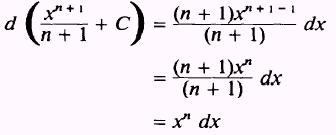Therefore,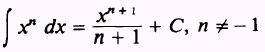EXAMPLE. EvaluateSOLUTION.*EXAMPLE. Evaluate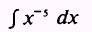SOLUTION.,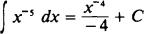CONSTANTS A constant may be written either before or after the integral sign. Formula.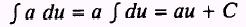PROOF: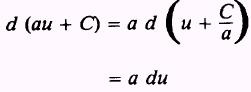Therefore,EXAMPLE. Evaluate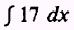SOLUTION:EXAMPLE: Evaluate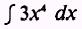SOLUTION: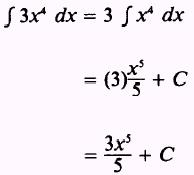Integrated Publishing, Inc. - A (SDVOSB) Service Disabled Veteran Owned Small Business